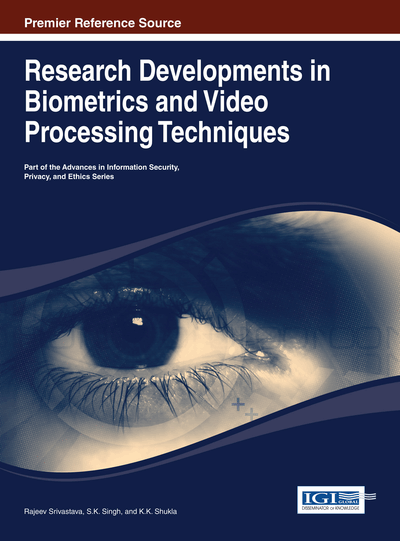# Daubechies Complex Wavelet-Based Computer Vision Applications

Manish Khare, Rajneesh Kumar Srivastava, Ashish Khare
DOI: 10.4018/978-1-4666-4868-5.ch007
OnDemand:
(Individual Chapters)
Available
\$29.50
No Current Special Offers

## Abstract

Many methods for computer vision applications have been developed using wavelet theory. Almost all of them are based on real-valued discrete wavelet transform. This chapter introduces two computer vision applications, namely moving object segmentation and moving shadow detection and removal, using Daubechies complex wavelet transform. Daubechies complex wavelet transform has advantages over discrete wavelet transform as it is approximately shift-invariant, has a better edge detection, and provides true phase information. Results after applying Daubechies complex wavelet transform on these two applications demonstrate that Daubechies complex wavelet transform-based methods provide better results than other real-valued wavelet transform-based methods, and it also demonstrates that Daubechies complex wavelet transform has the potential to be applied to other computer vision applications.
Chapter Preview
Top

## Introduction

In last two decades, many applications of wavelet theory have been emerged in the field of computer vision as an alternative to the well-known Fourier transform and its different variants. Wavelet transform was first introduced by Haar. The Haar wavelet function has compact support and it is an integer function. After era of Haar wavelet, a lot of work towards development of theoretical concepts of wavelet and its applications has been done. Now, various popular forms of wavelet transform exists such as, Continuous wavelet transform, Discrete wavelet transform and its variants, Hyper analytic wavelet transform, Complex wavelet transform, Integer wavelet transform and many more. These forms of wavelet transforms have been successfully applied on various computer vision applications like Denoising, Deblurring, Object Tracking, Object Classification, Activity Recognition, Shadow detection and removal etc.

Wavelet transforms are useful in image and video applications due to following reasons:

• 1.

Wavelet transform offers a simultaneous localization in time and frequency domain.

• 2.

Wavelet transform is computationally faster than Fourier transform.

• 3.

Wavelet transform is being able to separate fine details in an image.

• 4.

Multiresolution property of wavelet transform is helpful to analyze an image or video at several scales.

The Discrete Wavelet Transform (DWT) is most commonly used form of wavelet transform due to its compact representation, but this form of wavelet transform have several limitations such as – shift sensitivity, poor directional selectivity, poor edge detection and no phase information. Shift sensitivity means small shifts in the input signal causes major variations in the distribution of energy between discrete wavelet transform coefficients at different scales (Selesnick et al., 2005). This is an undesirable property, making DWT not suitable for those applications where object is present in shifted from e.g. object tracking, moving object segmentation etc. Figure 1 illustrates the shift sensitivity problem of discrete wavelet transform. Figure 1(a) represents signal having 512 sample points, in which two sample points take value 1 and the remaining take the value 0. Figure 1(b) shows the detailed wavelet coefficients of signal at several scales using real valued discrete wavelet transform. Here db4 wavelet has been used. From the Figure 1, it is clear that nature of real valued discrete wavelet coefficients in shifted signal as well as at multiple scales does not remain same. Thus, effect of applying same function will work in different way at different places and at difference scales.

In discrete wavelet transform, separable filtering along the rows and columns of an image produces four different images (LL, LH, HL, and HH) at each level. The LH subimage contains horizontal edges and HL subimage contains vertical edges, whereas HH subimage contains components from diagonal features. This means discrete wavelet transform has limited directional selectivity of four directions and due to this poor directionality, DWT have only limited directional edge detection characteristic.

Implementation of discrete wavelet transform uses real-valued wavelets and associated real filter-coefficients. Such a wavelet transform does not provide phase information. The information about the phase can be utilized in various image and signal processing applications, especially for certain class of coherent imaging systems. Amplitude-phase representation imparts greater robustness in signal and image processing applications. Unfortunately, since discrete real wavelet transform does not provide phase information at all, so such type of robustness in computer vision applications can’t be achieved using real valued discrete wavelet transform.

## Complete Chapter List

Search this Book:
Reset